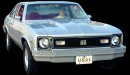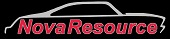Main Page | What's New? | Site Map | e-mailTransmission Codes This site is best viewed in 1024x768 resolution.Transmission identification may be determined by finding the transmission application code in the following tables. The transmission codes are found on the Protect-O-Plate and in the following locations: Muncie 3-speed = on the boss above the filler plug Muncie 4-speed = on the right side of the case at the right side of the cover flange Saginaw 3- and 4-speed = on the lower right side of the case next to the rear cover Powerglide = on the upper flange of the transmission housing converter opening Torque Drive = on the upper flange of the transmission housing converter opening TH200 = tag on the right side of the transmission extension TH350 (1972 and earlier)= on the upper flange of the transmission housing converter opening TH350 (1973 and later) = on the right vertical surface of the oil pan TH400 = on the upper flange of the transmission housing converter opening The codes on this page are not limited to Novas only. They cover all Chevrolet. The codes have been compiled from many sources to make this page as correct as possible. There is no guarantee that the codes are complete or accurate, therefore, I cannot accept any liability or responsibility for damages incurred from the use of this information. It is my intention to help you as much as possible in deciphering your Novas engine code. Good Luck and Happy Motoring!
Transmission Aplication Codes

1966 and earlier code example: C1012D

Plant Code / Trans Type Assembly Month Assembly Day Assembly Shift Muncie Suffix
A = Cleveland / Torque Drive
B = Cleveland / TH350 (1971-78)
B = Parma / TH350 (1979)
C = Cleveland / Powerglide
D = Parma / TH350
E = McKinnon / Powerglide
H = Muncie / 3-speed
K = McKinnon / 3-speed
M = Muncie / 3-speed
N = Muncie / 4-speed
O = Saginaw / Overdrive
P = Muncie / 4-speed M20, M21, M22
R = Saginaw / 4-speed (1964-69)
R = Muncie / 4-speed (1979)
S = Saginaw / 3-speed (1962-70)
S = Muncie / 3-speed (1971-79)
T = Toledo / Powerglide
W = Warner / 3-speed, Heavy Duty
X = Cleveland / TH350
Y = Cleveland / TH350
1 = January
2 = February
3 = March
4 = April
5 = May
6 = June
7 = July
8 = August
9 = September
10 = October
11 = November
12 = December
01 = 1st
02 = 2nd
03 = 3rd
04 = 4th
05 = 5th
06 = 6th
07 = 7th
08 = 8th
09 = 9th
10 = 10th
etc...
D = Day
N = Night
A = M20
B = M21
C = M22

1967 and later code example: C8A10D

Plant Code / Trans Type Year Assembly Month Assembly Day Assembly Shift Muncie Suffix
A = Cleveland / Torque Drive
B = Cleveland / TH350 (1971-78)
B = Parma / TH350 (1979)
C = Cleveland / Powerglide
D = Parma / TH350
E = McKinnon / Powerglide
H = Muncie / 3-speed
K = McKinnon / 3-speed
M = Muncie / 3-speed
N = Muncie / 4-speed
O = Saginaw / Overdrive
P = Muncie / 4-speed M20, M21, M22
R = Saginaw / 4-speed (1964-69)
R = Muncie / 4-speed (1979)
S = Saginaw / 3-speed (1962-70)
S = Muncie / 3-speed (1971-79)
T = Toledo / Powerglide
W = Warner / 3-speed, Heavy Duty
X = Cleveland / TH350
Y = Cleveland / TH350
7 = 1967, 1977
8 = 1968, 1978
9 = 1969, 1979
0 = 1970
1 = 1971
2 = 1972
3 = 1973
4 = 1974
5 = 1975
6 = 1976

A = January
B = February
C = March
D = April
E = May
H = June
K = July
M = August
P = September
R = October
S = November
T = December
01 = 1st
02 = 2nd
03 = 3rd
04 = 4th
05 = 5th
06 = 6th
07 = 7th
08 = 8th
09 = 9th
10 = 10th
etc...
D = Day
N = Night
A = M20
B = M21
C = M22

TH400 example: 68CA375

Year Engines / (Horsepower) Assembly Date (Julian)
65 = 1965
66 = 1966
67 = 1967
68 = 1968
69 = 1969
70 = 1970
71 = 1971
72 = 1972
73 = 1973
74 = 1974
75 = 1975
CA = 350, 396, 400 / (265, 325)
CB = 427, 454 / (390)
CC = 396 / (325)
CD = 327, 350, 400, 402 / (235, 250)
CE = 396 / (350)
CF = 402, 427, 454 / (335)
CG = 396, 454 / (265)
CH = 396, 427 / (390)
CJ = 396, 402, Truck 1/2- and 3/4-ton / (310)
CK = 327, 350 / (300) Corvette
CL = 427 / (400) Corvette
CM = 307
CP = Truck 1-ton
CQ = 427 / (335)
CR = 454
CS = 454
CT = Truck 1/2- and 3/4-ton
CW = 402
CX = 396, 427 / (375, 425, 430, 435 & COPO)
CY = 350, 396, 427, 454 / (245, 255, 425, 450)
CZ = 350 / (L-82)

Some codes may have a leading 'C' deleted.
example: 68A375
The Julian date starts January 1 of the previous year. A 1968 date of 375 would be January 10, 1968.
375 - 365 = 10

1968
Code Description Engines Assembly Plant
QP3-speed L4 S = Saganaw
QB3-speed L4, L6 S = Saganaw
HI4-speed V8 P = Muncie
R = Saganaw
YTPowerglide L4 C = Cleveland
T = Toledo
TBPowerglide L6 C = Cleveland
T = Toledo
UEPowerglide V8 C = Cleveland
T = Toledo

1969
Code Description Engines Assembly Plant
QP3-speed L4 S = Saganaw
QB3-speed L4, L6 S = Saganaw
HU4-speed V8 P = Muncie
R = Saganaw
IETorque Drive L4 A = Cleveland
ICTorque Drive L6 A = Cleveland
YTPowerglide L4 C = Cleveland
T = Toledo
VEPowerglide L6 C = Cleveland
T = Toledo
UEPowerglide V8 C = Cleveland
T = Toledo
ISTH350 L6 X = Cleveland
Y = Toledo
ITTH350 V8 X = Cleveland
Y = Toledo

1970
Code Description Engines Assembly Plant
RR3-speed all M = Muncie
WA4-speed V8 P = Muncie
ULTorque Drive L4, L6 A = Cleveland
VEPowerglide L6 C = Cleveland
T = Toledo
UEPowerglide V8 C = Cleveland
T = Toledo
GNTH350 L6 X = Cleveland
Y = Toledo
GSTH350 V8 X = Cleveland
Y = Toledo

1971
Code Description Engines Assembly Plant
R33-speed L6, V8 S = Muncie
WT4-speed V8 P = Muncie
TLTorque Drive L6 A = Cleveland
THPowerglide L6 C = Cleveland
E = McKinnon
TJPowerglide V8 C = Cleveland
E = McKinnon
HWTH350 V8 B = Cleveland
Y = Toledo

1972
Code Description Engines Assembly Plant
R33-speed L6, V8 S = Muncie
WC4-speed V8 P = Muncie
RBPowerglide L6 C = Cleveland
RKPowerglide V8 C = Cleveland
SBTH350 V8 B = Cleveland
Y = Toledo

1973
Code Description Engines Assembly Plant
TM3-speed L6, V8 S = Muncie
WC4-speed V8 P = Muncie
TRPowerglide L6 C = Cleveland
FATH350 V8 B = Cleveland
Y = Toledo

1974
Code Description Engines Assembly Plant
TM3-speed L6, V8 S = Muncie
WC4-speed V8 P = Muncie
TTTH350 L6 B = Cleveland
THTH350 V8 Y = Toledo

1975
Code Description Engines Assembly Plant
CH3-speed L6, V8 S = Muncie
WC4-speed V8 P = Muncie
TZTH350 L6 B = Cleveland
YATH350 V8 Y = Toledo

1976
Code Description Engines Assembly Plant
UX3-speed L6, V8 S = Muncie
UF4-speed V8 P = Muncie
TKTH350 L6 D = Parma
XETH350 V8 Y = Toledo

1977
Code Description Engines Assembly Plant
ZC3-speed L6, V8 S = Muncie
ZM4-speed V8 P = Muncie
WKTH350 L6 D = Parma
AGTH350 V8 Y = Toledo

1978
Code Description Engines Assembly Plant
UR 3-speed L6, V8 S = Muncie
ZM 4-speed V8 P = Muncie
5WZTH350 L6 D = Parma
AG TH350 V8 Y = Toledo

1979
Code Description Engines Assembly Plant
SF 3-speed L6, V8 S = Muncie
SR 4-speed V8 R = Muncie
6TCTH350 L6 B = Parma
6TATH350 V8 Y = ToledoFollow NovaResource on Instagram and YouTube If you would like your Nova featured on the NovaResource Instragram feed, send pictures and a short description to scott@novaresource.org